# Load Factor: What is it? (And How To Calculate It)

Contents

In electrical engineering, the Load Factor is defined as a ratio of the average load divided by the maximum (or peak) load in a given time of period. In other words, the Load Factor is the ratio of total energy (kWh) used over a specific period of time to the total possible energy available within that period (i.e. peak demand over that specific time period). Load Factor can be calculated on a daily, monthly, or yearly basis. The equation of Load Factor is;The Load Factor is used to measure the utilization rate (means the efficiency of usage of electrical energy). The value of the Load Factor is always less than one. Because the average load will always less than the maximum demand.

The high value of Load Factor means the load is using electrical energy more efficiently. High Load Factor gives more saving of electrical energy. And low Load Factor means the electricity used insufficiently compared to your maximum demand.

Improved load factor means to reduce the peak load demand. This will increase the value of the Load Factor and saves electrical energy. It will also reduce the average cost per unit (kWh). This procedure is also known as load balancing or peak saving.

Improved load factor means to reduce the peak load demand. This will increase the value of the Load Factor and saves electrical energy. It will also reduce the average cost per unit (kWh). This procedure is also known as load balancing or peak saving.

A low load factor means high maximum demand and low utilization rate. If the Load factor is very low as high peak demand, the capacity of electrical energy remains inactive for a long time. And this will increase the per-unit cost of electrical energy to the consumer. To reduce the peak demand, shift some load from peak time to non-peak time.

For generators or power plants, the Load Factor is an important factor to find the efficiency of the power plant. For power plants, the Load Factor is defined as a ratio of energy generated in a given time of period to the product of maximum load and number of hours of operation.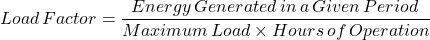## How to Calculate Load Factor?

The Load Factor is calculated by dividing the total consumption of electrical energy (kWh) for a given specific time of period to the product of maximum demand (kW) and the number of hours in that period.

The Load Factor can be calculated over any time of period. Generally, it is calculated based on a daily, weekly, monthly, or annual basis. The below equations show the Load Factor for a different time.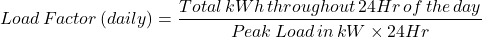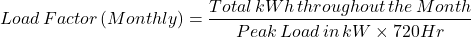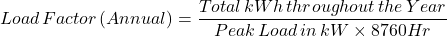Let’s calculate the Load Factor for the below condition. The above equations are multiplied by 100 to calculate the Load Factor in terms of percentage.

Monthly energy consumption is 36000 kWh and maximum demand of 100 kW.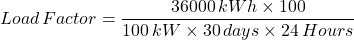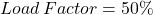Let’s take the same example but the maximum demand is reduced from 100 kW to 70 kW.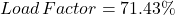So, the Load Factor is improved by reducing the maximum demand.

## How to Improve Load Factor?

The load factor is a ratio of average load to the maximum demand. Hence, it depends on the value of the average load and maximum demand. The consumer tries to keep the load factor as high as possible. Load Factor cannot be more than one as the average load is always less than the maximum demand.

The meaning of improving Load Factor means to increase the value of Load Factor. If the consumer tries to keep average load near the maximum demand, then the Load Factor will increase.

But instead of varying the average load, it is easy for consumers to reduce the maximum demand. The maximum demand can be reduced by shifting peak load to below-average load. The below figure shows the load curve for a day.